# Python中二维列表如何获取子区域元素的组成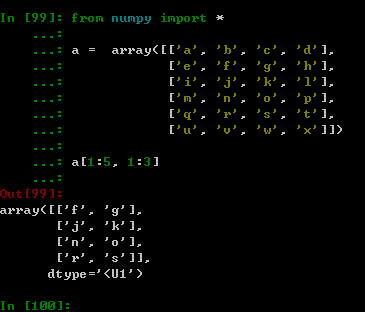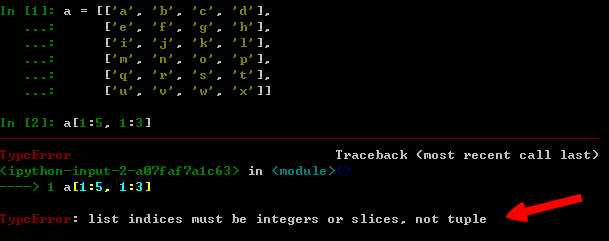```class Array:
"""实现__getitem__，支持序列获取元素、Slice等特性"""

def __init__(self, lst):
self.__coll = lst

def __repr__(self):
"""显示列表"""

return '{!r}'.format(self.__coll)

def __getitem__(self, key):
"""获取元素"""
slice1, slice2 = key
row1 = slice1.start
row2 = slice1.stop
col1 = slice2.start
col2 = slice2.stop
return [self.__coll[r][col1:col2] for r in range(row1, row2)]```

```a = Array([['a', 'b', 'c', 'd'],
['e', 'f', 'g', 'h'],
['i', 'j', 'k', 'l'],
['m', 'n', 'o', 'p'],
['q', 'r', 's', 't'],
['u', 'v', 'w', 'x']])

print(a[1:5, 1:3])```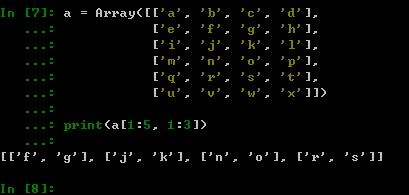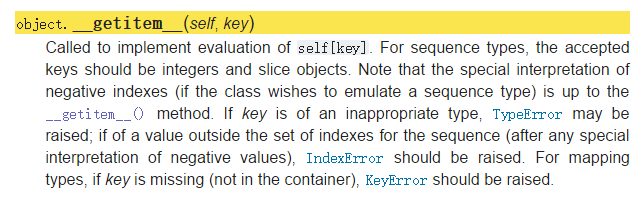```a = [['a', 'b', 'c', 'd'],
['e', 'f', 'g', 'h'],
['i', 'j', 'k', 'l'],
['m', 'n', 'o', 'p'],
['q', 'r', 's', 't'],
['u', 'v', 'w', 'x']]

sl = lambda row1, row2, col1, col2, lst:
[lst[r][col1:col2] for r in range(row1, row2)]

sl(1, 5, 1, 3, a)```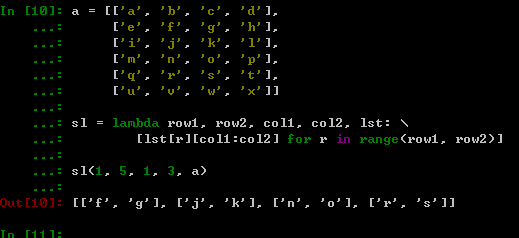Python正则替换字符串函数re.sub用法示例

python中实现迭代器（iterator）的方法示例

python django 访问静态文件出现404或500错误
djangostatic文件夹下面的内容方法不了出现404500错误需要查看自己的settings文件确保有一下内容importosPROJECT_ROOT=os.path.dirname(__file__)DEBUG=TrueSTATIC_URL='/static/'S

Copyright © 2010 Gimoo.Net. All Rights Rreserved  京ICP备05050695号